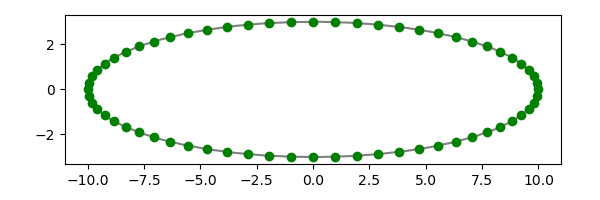# Uniform sampling from an ellipse

There is a simple way to randomly sample points from an ellipse, but it is not uniform.

Assume your ellipse is parameterized bywith t running from 0 to 2π. The naive approach would be to take uniform samples from t and stick them into the equations above.

Rather than looking at random sampling, this post will look at even sampling because it’s easier to tell visually whether even samples are correct. Random samples could be biased in a way that isn’t easy to see. We’ll use an ellipse with a= 10 and b = 3 because it’s easier to see the unevenness of the naive approach when the ellipse is more eccentric.

Let’s choose our t values by dividing the interval from 0 to 2π into 64 intervals. Here’s what we get when we push this up onto the ellipse.The spacing of the dots is not uniform, not on the scale of arc length. The spacing of the dots is uniform, but on the scale of our parameter t rather than on the geometrically relevant scale.

So how do you create evenly spaced dots on an ellipse? You unroll the ellipse into a line segment, sample from that segment, then roll the ellipse back up.

The previous post showed that the perimeter of an ellipse equals 4 E(1 – b²/a²) where E is the elliptic integral of the second kind. This tells us how long a segment we’ll get when we cut our ellipse and flatten it out to a segment. We take evenly spaced samples from the segment, then invert the arc length to project the points onto the ellipse.

If we redo our example with the procedure described above we get the following.Here’s how the dots in the plot above were computed. Describe the position of a point on the ellipse by the length of the arc from (0, a) to the point. Then you can find the corresponding parameter t with the following code.

    from scipy.special import ellipe, ellipeinc
from numpy import pi, sin, cos
from scipy.optimize import newton

def E_inverse(z, m):
em = ellipe(m)
t = (z/em)*(pi/2)
f = lambda y: ellipeinc(y, m) - z
r = newton(f, t)
return r

def t_from_length(length, a, b):
m = 1 - (b/a)**2
T = 0.5*pi - E_inverse(ellipe(m) - length/a, m)
return T


The same procedure applies to random samples. First randomly sample an interval as long as the perimeter of the ellipse to get a set of arc lengths. Then use t_from_length to get the values of t to stick into the parameterization of the ellipse.

I imagine there are more efficient ways to generate uniform samples from an ellipse, but this works. If this were the bottleneck in some application I’d try out other approaches.

## One thought on “Uniform sampling from an ellipse”

1. I think there is an easier and more efficient way. It is well known that you can generate points uniformly at random on the unit sphere by choosing X ~ MVN(0,I) and then forming X/||X||. The uniform distribution is preserved under linear transformations, so you can map the unit sphere to an ellipse in standard position by using L=diag(w1, w2, …, wd). For details and pictures, see https://blogs.sas.com/content/iml/2023/08/28/random-uniform-ellipse.html